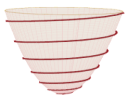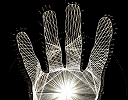Search IntMath
Close

450+ Math Lessons written by Math Professors and Teachers

5 Million+ Students Helped Each Year

1200+ Articles Written by Math Educators and Enthusiasts

Simplifying and Teaching Math for Over 23 Years

# IntMath Newsletter: Arc length of paraboloid spiral, brain injury to math genius

By Murray Bourne, 18 Jun 2014

18 Jun 2014

1. Arc length of a spiral around a paraboloid
2. Padgett - from brain injury to math genius
3. Thank you, volunteers!
4. Math puzzles
5. Final thought - Asking good questions

## 1. Arc length of a spiral around a paraboloidWhat is the length of a spiral around a paraboloid? This is an interesting application of integral calculus, involving an environmentally friendly cooker design. Arc length of a spiral around a paraboloid

## 2. Padgett - from brain injury to math geniusThis is a fascinating story. Jason Padgett was attacked by 2 men one night and savagely beaten. He ended up with severe concussion and an amazing new ability to "see the world through the lens of geometry". See the full story at: Brain Injury Turns Man Into Math Genius

Don't miss the gallery of his art while you're there.

## 3. Thank you, volunteers!

Last mail I asked for volunteers to help transcribing some calculus videos on IntMath.

Thank you very much to Abby, Rusty, Li-sa, Kok Soon, and Suzanne for helping out. Good job and much appreciated.

## 4. Math puzzles

The puzzle in the last IntMath Newsletter involved a bet between Brigette and Ric, concerning cars.

Correct answers with explanation were given by Tomas, Saikrishna and Michael, giving a good insight into the thought processes they used.

New math puzzle: A box contains 20 balls numbered 1 to 20. What is the probability that any two of these balls chosen from the box will have numbers that are relatively prime?

## 5. Final thought: Asking good questions is a vital skill

A lot of people who write to me have trouble articulating where their confusion lies. In class, a lot of students shy away from asking questions because they don't want to look stupid.

A common theme here is that students often don't have the skills to ask questions.

Dan Rothstein and Luz Santana in the book Make Just One Change: Teach Students to Ask Their Own Questions make the following crucial point:

The skill of being able to generate a wide range of questions and strategize about how to use them effectively is rarely, if ever, deliberately taught.

Asking good questions is essential for effective learning. When was the last time you were in a math class that taught how to ask good questions?

Until next time, enjoy whatever you learn.

### 6 Comments on “IntMath Newsletter: Arc length of paraboloid spiral, brain injury to math genius”

1. Tomas Garza says:

I assume the two balls are selected at random, without replacement. There are 20 x 19/2 = 190 possible pairs, and the number of pairs whose elements are relative primes may be found by direct enumeration to give 127. I used Mathematica to calculate these numbers, using built-in functions:

All possible pairs: sampleswor = Subsets[Range, {2}]
Pairs where the two elements are relative primes:
relprime = Select[sampleswor, CoprimeQ[Sequence @@ #] &]

Then, the probability is Length[relprime]/Length[sampleswor] = 0.668421

2. Patrick Lumumba says:

Out of the 20 numbers, there are 8 prime numbers. that is, 2,5, 5, 7, 11, 13, 17 and 19.
The probability of picking a prime number is given by:
P(prime no.)=8/20
=2/5
The probability of picking another prime number(having in mind that a prime number had been picked in the first picking) will be given by:
P(prime no.) =7/19
Therefore, the probability of picking two prime numbers will be: 2/5*7/19= 14/95

3. sudha says:

I am a teacher handling the paper Discrete mathematics. I have one question. How to make discrete mathematics class interesting?

4. Murray says:

Hi Sudha. Like any math subject that is potentially quite dry, it's important to help students realize the human (or creative) aspects of what they are learning, and also why they are learning it. A warm classroom environment that includes humor goes a long way, too.

These tips should help: https://www.intmath.com/blog/how-to-make-math-class-interesting

5. Nicos Mavrommatis says:

The number of pairs picked out of n balls is (n+1)n/2. For n=20 the sample space is 210 pairs. Each number makes 19 pairs with the others. The number of pairs relatively prime for each number is given below:

1,2,3, 4, 5,6, 7,8,9,10,11,12,13,14,15,16,17,18,19,20
I I I I I I I I I I I I I I I I I I I I
19+9+12+8+12+5+12+6+8+ 4+ 9+ 3+ 7+ 3+ 3+ 2+ 3+ 1+ 1+ 0= 127 of relatively prime pairs out of 210. So, the probability is:
P=127/210 or 0.6047

6. don miller says:

14/95

first draw 2/5
second draw 7/19

14/95 = 2/5 x 7/19

### Comment Preview

HTML: You can use simple tags like <b>, <a href="...">, etc.

To enter math, you can can either:

1. Use simple calculator-like input in the following format (surround your math in backticks, or qq on tablet or phone):
a^2 = sqrt(b^2 + c^2)
(See more on ASCIIMath syntax); or
2. Use simple LaTeX in the following format. Surround your math with $$ and $$.
$$\int g dx = \sqrt{\frac{a}{b}}$$
(This is standard simple LaTeX.)

NOTE: You can mix both types of math entry in your comment.